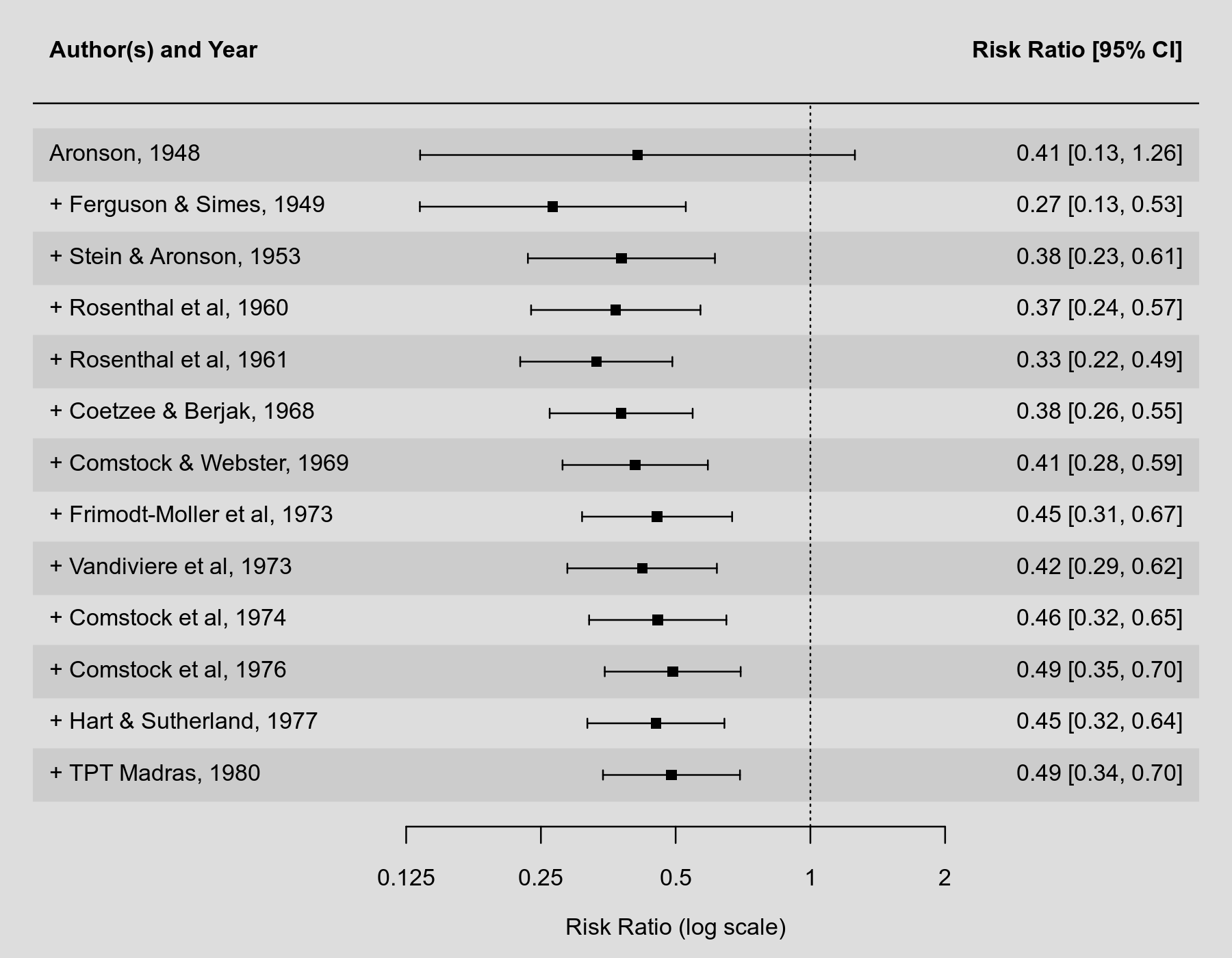#The metafor Package

A Meta-Analysis Package for R

### Sidebar

Action disabled: revisions
plots:cumulative_forest_plot

## Cumulative Forest Plot

### Description

A cumulative meta-analysis describes the accumulation of evidence (e.g., about the effectiveness of a particular treatment or intervention) as the available estimates are added to the analysis in (typically) chronological order (Chalmers & Lau, 1993; Lau et al., 1995). After fitting a model, for example with the rma() function, a cumulative meta-analysis can be conducted with the cumul() function. The results obtained that way can then be passed to the forest() function, which will draw a cumulative forest plot.

### Plot### Code

library(metafor)

### calculate (log) risk ratios and corresponding sampling variances
dat <- escalc(measure="RR", ai=tpos, bi=tneg, ci=cpos, di=cneg, data=dat.bcg)

### fit random-effects models
res <- rma(yi, vi, data=dat, slab=paste(author, year, sep=", "))

### cumulative meta-analysis (in the order of publication year)
tmp <- cumul(res, order=dat\$year)

### cumulative forest plot
forest(tmp, xlim=c(-4,2), at=log(c(0.125, 0.25, 0.5, 1, 2)),
atransf=exp, digits=c(2L,3L), cex=0.75, header="Author(s) and Year")

Chalmers, T. C., & Lau, J. (1993). Meta-analytic stimulus for changes in clinical trials. Statistical Methods in Medical Research, 2, 161–172.

Lau, J., Schmid, C. H., & Chalmers, T. C. (1995). Cumulative meta-analysis of clinical trials builds evidence for exemplary medical care. Journal of Clinical Epidemiology, 48, 45–57.

### Page Tools